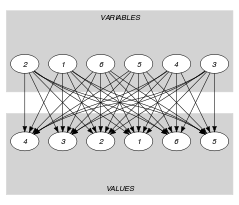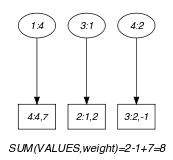## 5.420. weighted_partial_alldiff

Origin
Constraint

$\mathrm{𝚠𝚎𝚒𝚐𝚑𝚝𝚎𝚍}_\mathrm{𝚙𝚊𝚛𝚝𝚒𝚊𝚕}_\mathrm{𝚊𝚕𝚕𝚍𝚒𝚏𝚏}\left(\mathrm{𝚅𝙰𝚁𝙸𝙰𝙱𝙻𝙴𝚂},\mathrm{𝚄𝙽𝙳𝙴𝙵𝙸𝙽𝙴𝙳},\mathrm{𝚅𝙰𝙻𝚄𝙴𝚂},\mathrm{𝙲𝙾𝚂𝚃}\right)$

Synonyms

$\mathrm{𝚠𝚎𝚒𝚐𝚑𝚝𝚎𝚍}_\mathrm{𝚙𝚊𝚛𝚝𝚒𝚊𝚕}_\mathrm{𝚊𝚕𝚕𝚍𝚒𝚏𝚏𝚎𝚛𝚎𝚗𝚝}$, $\mathrm{𝚠𝚎𝚒𝚐𝚑𝚝𝚎𝚍}_\mathrm{𝚙𝚊𝚛𝚝𝚒𝚊𝚕}_\mathrm{𝚊𝚕𝚕𝚍𝚒𝚜𝚝𝚒𝚗𝚌𝚝}$, $\mathrm{𝚠𝚙𝚊}$.

Arguments
 $\mathrm{𝚅𝙰𝚁𝙸𝙰𝙱𝙻𝙴𝚂}$ $\mathrm{𝚌𝚘𝚕𝚕𝚎𝚌𝚝𝚒𝚘𝚗}\left(\mathrm{𝚟𝚊𝚛}-\mathrm{𝚍𝚟𝚊𝚛}\right)$ $\mathrm{𝚄𝙽𝙳𝙴𝙵𝙸𝙽𝙴𝙳}$ $\mathrm{𝚒𝚗𝚝}$ $\mathrm{𝚅𝙰𝙻𝚄𝙴𝚂}$ $\mathrm{𝚌𝚘𝚕𝚕𝚎𝚌𝚝𝚒𝚘𝚗}\left(\mathrm{𝚟𝚊𝚕}-\mathrm{𝚒𝚗𝚝},\mathrm{𝚠𝚎𝚒𝚐𝚑𝚝}-\mathrm{𝚒𝚗𝚝}\right)$ $\mathrm{𝙲𝙾𝚂𝚃}$ $\mathrm{𝚍𝚟𝚊𝚛}$
Restrictions
 $\mathrm{𝚛𝚎𝚚𝚞𝚒𝚛𝚎𝚍}$$\left(\mathrm{𝚅𝙰𝚁𝙸𝙰𝙱𝙻𝙴𝚂},\mathrm{𝚟𝚊𝚛}\right)$ $|\mathrm{𝚅𝙰𝙻𝚄𝙴𝚂}|>0$ $\mathrm{𝚛𝚎𝚚𝚞𝚒𝚛𝚎𝚍}$$\left(\mathrm{𝚅𝙰𝙻𝚄𝙴𝚂},\left[\mathrm{𝚟𝚊𝚕},\mathrm{𝚠𝚎𝚒𝚐𝚑𝚝}\right]\right)$ $\mathrm{𝚒𝚗}_\mathrm{𝚊𝚝𝚝𝚛}$$\left(\mathrm{𝚅𝙰𝚁𝙸𝙰𝙱𝙻𝙴𝚂},\mathrm{𝚟𝚊𝚛},\mathrm{𝚅𝙰𝙻𝚄𝙴𝚂},\mathrm{𝚟𝚊𝚕}\right)$ $\mathrm{𝚍𝚒𝚜𝚝𝚒𝚗𝚌𝚝}$$\left(\mathrm{𝚅𝙰𝙻𝚄𝙴𝚂},\mathrm{𝚟𝚊𝚕}\right)$
Purpose

All variables of the $\mathrm{𝚅𝙰𝚁𝙸𝙰𝙱𝙻𝙴𝚂}$ collection that are not assigned to value $\mathrm{𝚄𝙽𝙳𝙴𝙵𝙸𝙽𝙴𝙳}$ must have pairwise distinct values from the $\mathrm{𝚟𝚊𝚕}$ attribute of the $\mathrm{𝚅𝙰𝙻𝚄𝙴𝚂}$ collection. In addition $\mathrm{𝙲𝙾𝚂𝚃}$ is the sum of the $\mathrm{𝚠𝚎𝚒𝚐𝚑𝚝}$ attributes associated with the values assigned to the variables of $\mathrm{𝚅𝙰𝚁𝙸𝙰𝙱𝙻𝙴𝚂}$. Within the $\mathrm{𝚅𝙰𝙻𝚄𝙴𝚂}$ collection, value $\mathrm{𝚄𝙽𝙳𝙴𝙵𝙸𝙽𝙴𝙳}$ must be explicitly defined with a weight of 0.

Example
$\left(\begin{array}{c}〈4,0,1,2,0,0〉,0,\hfill \\ 〈\begin{array}{cc}\mathrm{𝚟𝚊𝚕}-0\hfill & \mathrm{𝚠𝚎𝚒𝚐𝚑𝚝}-0,\hfill \\ \mathrm{𝚟𝚊𝚕}-1\hfill & \mathrm{𝚠𝚎𝚒𝚐𝚑𝚝}-2,\hfill \\ \mathrm{𝚟𝚊𝚕}-2\hfill & \mathrm{𝚠𝚎𝚒𝚐𝚑𝚝}--1,\hfill \\ \mathrm{𝚟𝚊𝚕}-4\hfill & \mathrm{𝚠𝚎𝚒𝚐𝚑𝚝}-7,\hfill \\ \mathrm{𝚟𝚊𝚕}-5\hfill & \mathrm{𝚠𝚎𝚒𝚐𝚑𝚝}--8,\hfill \\ \mathrm{𝚟𝚊𝚕}-6\hfill & \mathrm{𝚠𝚎𝚒𝚐𝚑𝚝}-2\hfill \end{array}〉,8\hfill \end{array}\right)$

The $\mathrm{𝚠𝚎𝚒𝚐𝚑𝚝𝚎𝚍}_\mathrm{𝚙𝚊𝚛𝚝𝚒𝚊𝚕}_\mathrm{𝚊𝚕𝚕𝚍𝚒𝚏𝚏}$ constraint holds since:

• No value, except value $\mathrm{𝚄𝙽𝙳𝙴𝙵𝙸𝙽𝙴𝙳}=0$, is used more than once.

• $\mathrm{𝙲𝙾𝚂𝚃}=8$ is equal to the sum of the weights 2, $-1$ and 7 of the values 1, 2 and 4 assigned to the variables of $\mathrm{𝚅𝙰𝚁𝙸𝙰𝙱𝙻𝙴𝚂}=〈4,0,1,2,0,0〉$.

Typical
 $|\mathrm{𝚅𝙰𝚁𝙸𝙰𝙱𝙻𝙴𝚂}|>0$ $\mathrm{𝚊𝚝𝚕𝚎𝚊𝚜𝚝}$$\left(1,\mathrm{𝚅𝙰𝚁𝙸𝙰𝙱𝙻𝙴𝚂},\mathrm{𝚄𝙽𝙳𝙴𝙵𝙸𝙽𝙴𝙳}\right)$ $|\mathrm{𝚅𝙰𝚁𝙸𝙰𝙱𝙻𝙴𝚂}|\le |\mathrm{𝚅𝙰𝙻𝚄𝙴𝚂}|+2$
Symmetries
• Items of $\mathrm{𝚅𝙰𝚁𝙸𝙰𝙱𝙻𝙴𝚂}$ are permutable.

• Items of $\mathrm{𝚅𝙰𝙻𝚄𝙴𝚂}$ are permutable.

• All occurrences of two distinct values in $\mathrm{𝚅𝙰𝚁𝙸𝙰𝙱𝙻𝙴𝚂}.\mathrm{𝚟𝚊𝚛}$ or $\mathrm{𝚅𝙰𝙻𝚄𝙴𝚂}.\mathrm{𝚟𝚊𝚕}$ that are both different from $\mathrm{𝚄𝙽𝙳𝙴𝙵𝙸𝙽𝙴𝙳}$ can be swapped; all occurrences of a value in $\mathrm{𝚅𝙰𝚁𝙸𝙰𝙱𝙻𝙴𝚂}.\mathrm{𝚟𝚊𝚛}$ or $\mathrm{𝚅𝙰𝙻𝚄𝙴𝚂}.\mathrm{𝚟𝚊𝚕}$ that is different from $\mathrm{𝚄𝙽𝙳𝙴𝙵𝙸𝙽𝙴𝙳}$ can be renamed to any unused value that is also different from $\mathrm{𝚄𝙽𝙳𝙴𝙵𝙸𝙽𝙴𝙳}$.

Arg. properties

Functional dependency: $\mathrm{𝙲𝙾𝚂𝚃}$ determined by $\mathrm{𝚅𝙰𝚁𝙸𝙰𝙱𝙻𝙴𝚂}$ and $\mathrm{𝚅𝙰𝙻𝚄𝙴𝚂}$.

Usage

In his PhD thesis [Thiel04], Sven Thiel describes the following three potential scenarios of the $\mathrm{𝚠𝚎𝚒𝚐𝚑𝚝𝚎𝚍}_\mathrm{𝚙𝚊𝚛𝚝𝚒𝚊𝚕}_\mathrm{𝚊𝚕𝚕𝚍𝚒𝚏𝚏}$ constraint:

• Given a set of tasks (i.e., the items of the $\mathrm{𝚅𝙰𝚁𝙸𝙰𝙱𝙻𝙴𝚂}$ collection), assign to each task a resource (i.e., an item of the $\mathrm{𝚅𝙰𝙻𝚄𝙴𝚂}$ collection). Except for the resource associated with value $\mathrm{𝚄𝙽𝙳𝙴𝙵𝙸𝙽𝙴𝙳}$, every resource can be used at most once. The cost of a resource is independent from the task to which the resource is assigned. The cost of value $\mathrm{𝚄𝙽𝙳𝙴𝙵𝙸𝙽𝙴𝙳}$ is equal to 0. The total cost $\mathrm{𝙲𝙾𝚂𝚃}$ of an assignment corresponds to the sum of the costs of the resources effectively assigned to the tasks. Finally we impose an upper bound on the total cost.

• Given a set of persons (i.e., the items of the $\mathrm{𝚅𝙰𝚁𝙸𝙰𝙱𝙻𝙴𝚂}$ collection), select for each person an offer (i.e., an item of the $\mathrm{𝚅𝙰𝙻𝚄𝙴𝚂}$ collection). Except for the offer associated with value $\mathrm{𝚄𝙽𝙳𝙴𝙵𝙸𝙽𝙴𝙳}$, every offer should be selected at most once. The profit associated with an offer is independent from the person that selects the offer. The profit of value $\mathrm{𝚄𝙽𝙳𝙴𝙵𝙸𝙽𝙴𝙳}$ is equal to 0. The total benefit $\mathrm{𝙲𝙾𝚂𝚃}$ is equal to the sum of the profits of the offers effectively selected. In addition we impose a lower bound on the total benefit.

• The last scenario deals with an application to an over-constraint problem involving the $\mathrm{𝚊𝚕𝚕𝚍𝚒𝚏𝚏𝚎𝚛𝚎𝚗𝚝}$ constraint. Allowing some variables to take an "undefined" value is done by setting all weights of all the values different from $\mathrm{𝚄𝙽𝙳𝙴𝙵𝙸𝙽𝙴𝙳}$ to 1. As a consequence all variables assigned to a value different from $\mathrm{𝚄𝙽𝙳𝙴𝙵𝙸𝙽𝙴𝙳}$ will have to take distinct values. The $\mathrm{𝙲𝙾𝚂𝚃}$ variable allows to control the number of such variables.

Remark

It was shown in [Thiel04] that, finding out whether the $\mathrm{𝚠𝚎𝚒𝚐𝚑𝚝𝚎𝚍}_\mathrm{𝚙𝚊𝚛𝚝𝚒𝚊𝚕}_\mathrm{𝚊𝚕𝚕𝚍𝚒𝚏𝚏}$ constraint has a solution or not is NP-hard. This was achieved by reduction from subset sum.

Algorithm

A filtering algorithm is given in [Thiel04]. After showing that, deciding whether the $\mathrm{𝚠𝚎𝚒𝚐𝚑𝚝𝚎𝚍}_\mathrm{𝚙𝚊𝚛𝚝𝚒𝚊𝚕}_\mathrm{𝚊𝚕𝚕𝚍𝚒𝚏𝚏}$ has a solution is NP-complete, [Thiel04] gives the following results of his filtering algorithm with respect to consistency under the 3 scenarios previously described:

• For scenario 1, if there is no restriction of the lower bound of the $\mathrm{𝙲𝙾𝚂𝚃}$ variable, the filtering algorithm achieves arc-consistency for all variables of the $\mathrm{𝚅𝙰𝚁𝙸𝙰𝙱𝙻𝙴𝚂}$ collection (but not for the $\mathrm{𝙲𝙾𝚂𝚃}$ variable itself).

• For scenario 2, if there is no restriction of the upper bound of the $\mathrm{𝙲𝙾𝚂𝚃}$ variable, the filtering algorithm achieves arc-consistency for all variables of the $\mathrm{𝚅𝙰𝚁𝙸𝙰𝙱𝙻𝙴𝚂}$ collection (but not for the $\mathrm{𝙲𝙾𝚂𝚃}$ variable itself).

• Finally, for scenario 3, the filtering algorithm achieves arc-consistency for all variables of the $\mathrm{𝚅𝙰𝚁𝙸𝙰𝙱𝙻𝙴𝚂}$ collection as well as for the $\mathrm{𝙲𝙾𝚂𝚃}$ variable.

Keywords
Arc input(s)

$\mathrm{𝚅𝙰𝚁𝙸𝙰𝙱𝙻𝙴𝚂}$ $\mathrm{𝚅𝙰𝙻𝚄𝙴𝚂}$

Arc generator
$\mathrm{𝑃𝑅𝑂𝐷𝑈𝐶𝑇}$$↦\mathrm{𝚌𝚘𝚕𝚕𝚎𝚌𝚝𝚒𝚘𝚗}\left(\mathrm{𝚟𝚊𝚛𝚒𝚊𝚋𝚕𝚎𝚜},\mathrm{𝚟𝚊𝚕𝚞𝚎𝚜}\right)$

Arc arity
Arc constraint(s)
 $•\mathrm{𝚟𝚊𝚛𝚒𝚊𝚋𝚕𝚎𝚜}.\mathrm{𝚟𝚊𝚛}\ne \mathrm{𝚄𝙽𝙳𝙴𝙵𝙸𝙽𝙴𝙳}$ $•\mathrm{𝚟𝚊𝚛𝚒𝚊𝚋𝚕𝚎𝚜}.\mathrm{𝚟𝚊𝚛}=\mathrm{𝚟𝚊𝚕𝚞𝚎𝚜}.\mathrm{𝚟𝚊𝚕}$
Graph property(ies)
 $•$$\mathrm{𝐌𝐀𝐗}_\mathrm{𝐈𝐃}$$\le 1$ $•$$\mathrm{𝐒𝐔𝐌}$$\left(\mathrm{𝚅𝙰𝙻𝚄𝙴𝚂},\mathrm{𝚠𝚎𝚒𝚐𝚑𝚝}\right)=\mathrm{𝙲𝙾𝚂𝚃}$

Graph model

Parts (A) and (B) of Figure 5.420.1 respectively show the initial and final graph associated with the Example slot. Since we also use the $\mathrm{𝐒𝐔𝐌}$ graph property we show the vertices of the final graph from which we compute the total cost in a box.

##### Figure 5.420.1. Initial and final graph of the $\mathrm{𝚠𝚎𝚒𝚐𝚑𝚝𝚎𝚍}_\mathrm{𝚙𝚊𝚛𝚝𝚒𝚊𝚕}_\mathrm{𝚊𝚕𝚕𝚍𝚒𝚏𝚏}$ constraint(a) (b)